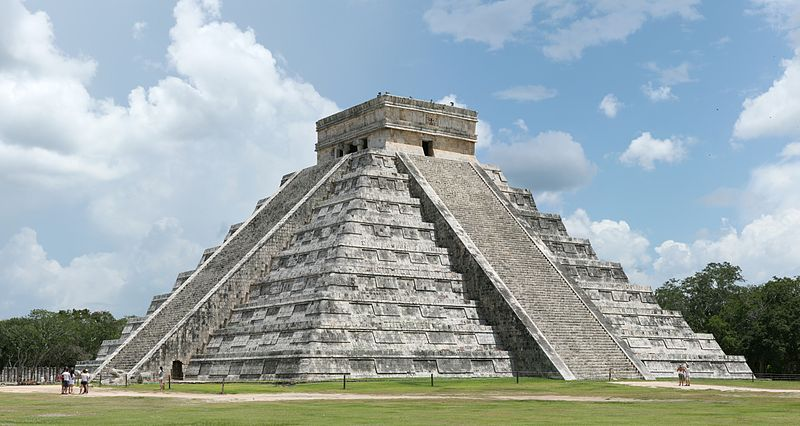# Climbing the steps of El Castillo

Alignments to Content Standards: 7.RP.A 6.RP.A

Chichén Itzá was a Mayan city in what is now Mexico. The picture below shows El Castillo, also known as the pyramid of Kukulcán, which is a pyramid located in the ruins of Chichén Itzá.The temple at the top of the pyramid is approximately 24 meters above the ground, and there are 91 steps leading up to the temple. How high above the ground would you be if you were standing on the 50th step?

## IM Commentary

The purpose of this task is for students to solve a straight-forward problem involving a proportional relationship in a context. In order to solve the problem, students must assume that the steps are of uniform height, which looks reasonable given the picture.

Two different and important approaches to the problem are presented: calculating a unit rate (height per step) and writing an equation (the height of the 50th step is $\frac{50}{91}$ the height of the pyramid). The first of these approaches falls under the 6th grade ratio and proportion standards while the second aligns better with the 7th grade. The main goal of the third approach, using Egyptian fractions, is to provide an example of calculating fluently. The methods of the first two solutions are vital for a deep understanding of proportional reasoning.

The numbers make this an advanced 6th grade task. It could be used as a transition from 6th to 7th grade expectations for work with ratios and proportional relationships, or it could be used as an assessment task in 7th grade (either formative or summative, depending on the time and way it is used). If used in an instructional setting, the teacher could ask the students to estimate before they compute. Since there are 91 steps and we want to know about the 50th step, we know that the height should be a little more than half of the total 24 meters in height.

This task could also be used to transition to the 8th grade standard on using similar triangles to prove that the slope of a line is well-defined. Looking at the cross-section of this pyramid and the similar triangles that we are essentially asking about would be a useful extension task:The image is courtesy Wikimedia Commons:

http://en.wikipedia.org/wiki/File:Chichen_Itza_3.jpg

## Solutions

Solution: 1

There are 91 steps needed to get you to the top, which is 24 meters high. So each step is about $24\div91$ meters tall. Since

$$24\div91=\frac{24}{91}\approx 0.264$$

we estimate that each step is about 0.264 m or 26.4 cm tall on average. Since

$$50\times \frac{24}{91}\approx 13.2$$

we'd expect to be about 13 meters above the ground if we stood on the 50th step.

Solution: 2

Assuming the steps are all the same size, the ratio of the height, $h$, of the 50th step to the height of the 91st step will be 50:91. So we know that

$$\frac{50}{91}=\frac{h}{24}$$

If we multiply both sides of this equation by 24, we get that

$$h=24\times\frac{50}{91}\approx 13.2$$

So the 50th step is about 13 meters off of the ground.

Solution: The solution for people who like Egyptian Fractions

Half of 91 is 45.5. One tenth of one half (or one twentieth) of 91 is 4.55. These add up to 50.05 and tell us that the 50th step is about $\frac{1}{2} + \frac{1}{20}$ of the way up the structure. The top is 24 meters. So we can estimate the height of the 50th step by adding $\frac12$ of 24 which is 12 and $\frac{1}{20}$ of 24 which is 1.2. Since $$12+1.2 = 13.2$$ we know we are about 13 meters above the ground.